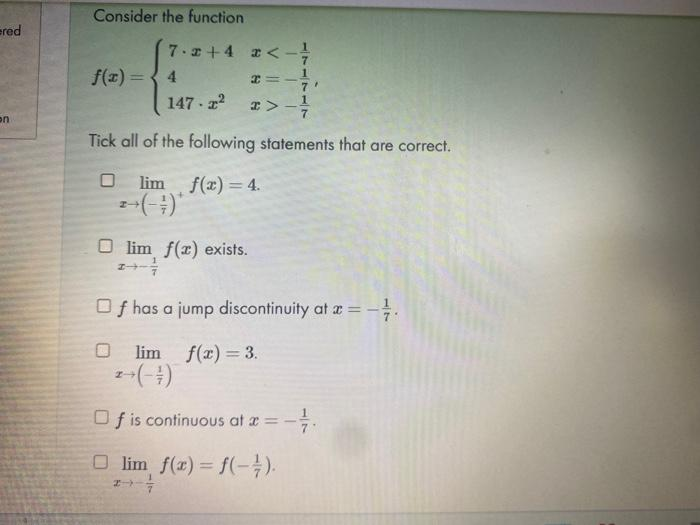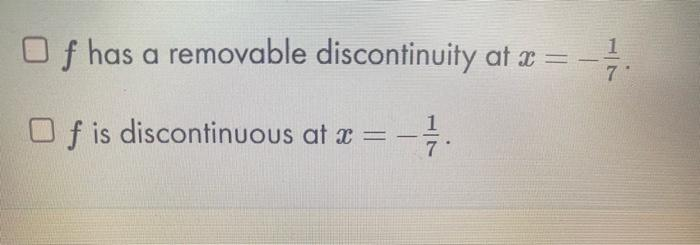Home / Expert Answers / Calculus / consider-the-function-f-x-left-begin-array-ll-7-cdot-x-4-x-frac-1-7-end-array-righ-pa287

# (Solved): Consider the function $f(x)=\left\{\begin{array}{ll} 7 \cdot x+4 & x-\frac{1}{7} \end{array}\righ ...Consider the function \[ f(x)=\left\{\begin{array}{ll} 7 \cdot x+4 & x<-\frac{1}{7} \\ 4 & x=-\frac{1}{7} \\ 147 \cdot x^{2} & x>-\frac{1}{7} \end{array}\right.$ Tick all of the following statements that are correct. $\lim _{x \rightarrow\left(-\frac{1}{7}\right)^{+}} f(x)=4$ $$\lim _{x \rightarrow-\frac{1}{7}} f(x)$$ exists. $$f$$ has a jump discontinuity at $$x=-\frac{1}{7}$$. $$\lim _{x \rightarrow\left(-\frac{1}{7}\right)} f(x)=3$$ $$f$$ is continuous at $$x=-\frac{1}{7}$$. $\lim _{x \rightarrow-\frac{1}{7}} f(x)=f\left(-\frac{1}{7}\right)$ $$f$$ has a removable discontinuity at $$x=-\frac{1}{7}$$. $$f$$ is discontinuous at $$x=-\frac{1}{7}$$.

We have an Answer from Expert